# Texas Go Math Grade 3 Lesson 9.5 Answer Key Multiply 2-Digit by 1-Digit Numbers

Refer to our Texas Go Math Grade 3 Answer Key Pdf to score good marks in the exams. Test yourself by practicing the problems from Texas Go Math Grade 3 Lesson 9.5 Answer Key Multiply 2-Digit by 1-Digit Numbers.

## Texas Go Math Grade 3 Lesson 9.5 Answer Key Multiply 2-Digit by 1-Digit Numbers

Essential Question
How can you use strategies to multiply a 2-digit number by a 1-digit number?
Multiply the 1 digits together first. Cross-multiply by multiplying diagonal digits, then add the two sums together. Multiply the 2-digit numbers.

Explanation:
Here is one way that you can speed up the process of 2-digit by 1-digit multiplication:
Multiply the 1 digits together first. Cross-multiply by multiplying diagonal digits, then add the two sums together. Multiply the 2-digit numbers.

Unlock the Problem
A Thoroughbred racehorse can run at speeds of up to 60 feet per second. During practice, Celia’s horse runs at a speed of 36 feet per second. How far does her horse run in 3 seconds?

• Underline important information.
• Is there information you will not use? If so, cross out the information.

Example 1.
Multiply. 3 × 36So, Celia’s racehorse runs ___ feet in 3 seconds.
So, Celia’s racehorse runs 108 feet in 3 seconds.

Explanation:
Number of feet Celia’s horse runs per second = 36.
Distance her horse runs in 3 seconds = 3 × Number of feet Celia’s horse runs per second
= 3 × 36
= (3 × 10) + (3 × 10) + (3 × 10) + (3 × 6)
= 30 + 30 + 30 + 18
= 60 + 30 + 18
= 90 + 18
= 108 feet.

Math Talk
Mathematical Processes
Look at Step 1. Explain how the blocks show the regrouping of the 18 ones.

Example 2.
Multiply. 8 × 22So, 8 × 22 = ____.

Math talk
Mathematical Processes
Why is 8 × 2 tens recorded as 160 and not as 16?
8 × 2 tens is recorded as 160 and not as 16 because 8 × 2 tens = 8 × 20 = 160 not 16.

Explanation:
8 × 2 tens = 8 × (2 × 10)
= 8 × 20
Multiply tens and ones place.
= 160.

Share and Show
Question 1.
Use the model to find the product.Explanation:
2 × 36 = (2 × 3 tens) + (2 × 6 ones)
= [2 ×(3 × 10)]+ [2 × (6 × 1)]
= (2 × 30) + (2 × 6)
= 60 + 12
= 72.

Find the product. Tell which strategy you used.
Question 2.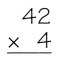Strategy used is multiplication and addition to find the product.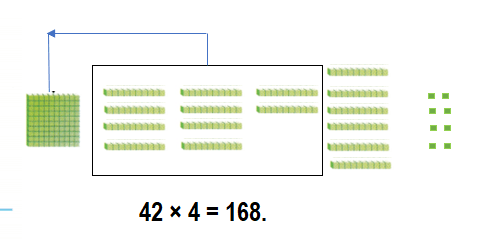Explanation:
42 × 4 = (40 × 4) + (2 × 4)
= 160 + 8
= 168.

Question 3.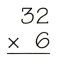Strategy used is multiplication and addition to find the product.Explanation:
32 × 6 = (30 × 6) + (2 × 6)
= 180 + 12
= 192.

Question 4.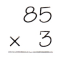Strategy used is multiplication and addition to find the product.Explanation:
85 × 3 = (80 × 3) + (5 × 3)
= 240 + 15
= 255.

Question 5.Strategy used is multiplication and addition to find the product.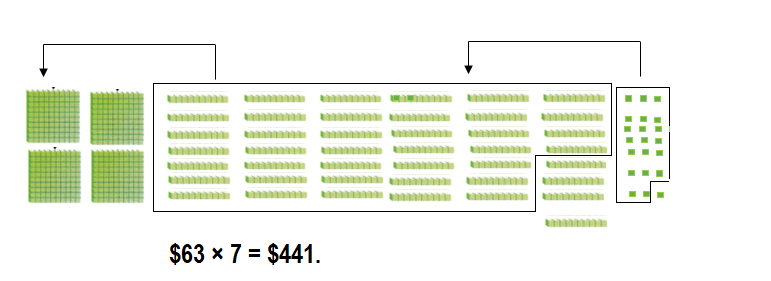Explanation:
$63 × 7 = ($60 × 7) + ($3 × 7) =$420 + $21 =$441.

Math Talk
Mathematical Processes
Describe the steps for using place value and regrouping to find 3 × 78.
Steps for using place value and regrouping to find 3 × 78:
1. Multiply the tens place and record the value.
2. Multiply the ones place and record the value.
3. Do add the both vales.
3 × 78 = 234.

Explanation:
Steps for using place value and regrouping to find 3 × 78:
1. Multiply the tens place and record the value:
3 × 70 = 210.
2. Multiply the ones place and record the value:
3 × 8 = 24.
3. Do add the both vales:
=> (3 × 70) + (3 × 8)
= 210 + 24
= 234.Problem Solving
Use the table for 6-8.
Question 6.
How far can a desert cottontail run in 9 seconds?Distance desert cottontail runs 9 seconds = 198 feet.

Explanation:
Distance desert cottontail runs per second = 22 feet.
Distance desert cottontail runs 9 seconds = 9 × Distance desert cottontail runs per second
= 9 × 22
= (9 × 10) + (9 × 10) + (9 × 2)
= 90 + 90 + 18
= 180 + 18
= 198 feet.

Question 7.
Analyze A black-tailed jackrabbit hops about 7 feet in a single hop. How far can it hop in 5 seconds?Distance black-tailed jackrabbit hops in a 5 seconds = 35 feet.

Explanation:
Distance black-tailed jackrabbit hops in a single hop  per second = 7 feet.
Distance black-tailed jackrabbit hops in a 5 seconds = 5 × Distance black-tailed jackrabbit hops in a single hop  per second
= 5 × 7
= 35 feet.

Question 8.
H.O.T. Multi-Step At the speeds shown, how much farther could a black-tailed jackrabbit run than a desert cottontail in 7 seconds?
203 feet farther a black-tailed jackrabbit run than a desert cottontail in 7 seconds.

Explanation:
Distance a black-tailed jackrabbit run in a second = 51.
Distance a black-tailed jackrabbit run in 7 seconds = 7 × Distance a black-tailed jackrabbit run in a second
= 7 × 51
= (7 × 10) + (7 × 10) + (7 × 10) + (7 × 10) + (7 × 10) + (7 × 1)
= 70 + 70 + 70 + 70  + 70 + 7
= 140 + 70 + 70  + 70 + 7
= 210 + 70  + 70 + 7
= 280 + 70 + 7
= 350 + 7
= 357 feet.
Distance a desert cottontail run in a second = 22.
Distance a desert cottontail run in 7 seconds = 7 × Distance a desert cottontail run in a second
= 7 × 22
=(7 × 10) + (7 × 10) +(7 × 2)
= 70 + 70 + 14
= 140 + 14
= 154 feet.
Difference:
Distance a black-tailed jackrabbit run in 7 seconds – Distance a desert cottontail run in 7 seconds
= 357 – 154
= 203 feet.

Question 9.
Multi-Step Mr. Wright bought a 3-pound bag of cat food and a 5 pound bag of dog food, There are 16 ounces in each pound. How many ounces of pet food did Mr. Wright buy?
Number of ounces of pet food Mr. Wright buy = 128.

Explanation:
Number of pounds bag of cat food Mr. Wright bought = 3.
Number of pounds bag of dog food Mr. Wright bought = 5.
Number of ounces in each pound = 16.
Number of ounces are in bag of cat food Mr. Wright bought = Number of pounds bag of cat food Mr. Wright bought ×Number of ounces in each pound
= 3 × 16
=(3 × 10 + (3 × 6)
= 30 + 18
= 48.
Number of ounces are in bag of dog food Mr. Wright bought = Number of pounds bag of dog food Mr. Wright bought × Number of ounces in each pound
= 5 × 16
=(5 × 10) + (5 × 6)
= 50 + 30
= 80.
Number of ounces of pet food Mr. Wright buy = Number of ounces are in bag of cat food Mr. Wright bought + Number of ounces are in bag of dog food Mr. Wright bought
= 48 + 80
= 128.

Question 10.
H.O.T. Reasoning The sum of two numbers is 31. The product of the two numbers is 150. What are the numbers?
The numbers are 6 and 25.

Explanation:
The sum of two numbers is 31.
The product of the two numbers is 150.
Let the numbers be X and Y.
Multiples of 150:
3 × 50 = 150. => 3 + 50 = 53.
5 × 30 = 150. => 5 + 30 = 35.
6 × 25 = 150. => 6 + 25 = 31.
10 × 15 = 150. => 10 + 15 = 25.

Question 11.
Write Math 6 × 87 is greater than 5 × 87. How much greater? Explain how you know without multiplying.
Difference = 87.
Without multiply:
(6 × 87) – (5 × 87) = difference of multiple (6 – 5) × 87 = 1 × 87 = 87.

Explanation:
Product of 6 × 87 = (6 × 10) + ( 6 × 10) + (6 × 10) + ( 6 × 10) + (6 × 10) + ( 6 × 10) + (6 × 10) + ( 6 × 10) + (6 × 7)
= 60 + 60 + 60 + 60 + 60 + 60 + 60 + 60 + 42
= 120 + 60 + 60 + 60 + 60 + 60 + 60 + 42
= 180 + 60 + 60 + 60 + 60 + 60 + 42
= 240 + 60 + 60 + 60 + 60 + 42
= 300 + 60 + 60 + 60 + 42
= 360 + 60 + 60 + 42
= 420 + 60 + 42
= 480 + 42
= 522.
Product of 5 × 87 = (5 × 10) + (5 × 10) + (5 × 10) + (5 × 10) + (5 × 10) + (5 × 10) + (5 × 10) + (5 × 10) + (5 × 7)
= 50 + 50 + 50 + 50 + 50 + 50 + 50 + 50 + 35
= 100 + 50 + 50 + 50 + 50 + 50 + 50 + 35
= 150 + 50 + 50 + 50 + 50 + 50 + 35
= 200 + 50 + 50 + 50 + 50 + 35
= 250 + 50 + 50 + 50 + 35
= 300 + 50 + 50 + 35
= 350 + 50 + 35
= 400 + 35
= 435.
Difference:
Product of 6 × 87 – Product of 5 × 87
= 522 – 435
= 87.

Without multiply:
(6 × 87) – (5 × 87) = difference of multiple (6 – 5) × 87 = 1 × 87 = 87.

Fill in the bubble for the correct answer. Use a strategy to solve.
Question 12.
Clayton bought 7 boxes of markers for an art project. There are 12 markers in each box. How many markers did he buy?
(A) 84
(B) 14
(C) 19
(D) 74
Number of markers he buy = 84.
(A) 84.

Explanation:
Number of boxes of markers for an art project Clayton bought = 7.
Number of markers in each box = 12.
Number of markers he buy = Number of boxes of markers for an art project Clayton bought  × Number of markers in each box
= 7 × 12
= (7 × 10) + ( 7 × 2)
= 70 + 14
= 84.

Question 13.
Apply Alana has been on the swim team for 3 years. She practices 26 weeks a year. How many weeks has she practiced?
(A) 68
(B) 618
(C) 78
(D) 6,018
Number of weeks she practiced = 78.
(C) 78.

Explanation:
Number of years Alana has been on the swim team = 3.
Number of weeks a year she practices = 26.
Number of weeks she practiced = Number of years Alana has been on the swim team × Number of weeks a year she practices
= 3 × 26
= (3 × 10) + (3 × 10) +(3 × 6)
= 30 + 30 + 18
= 60 + 18
= 78.

Question 14.
Multi-Step At Huff and Puff Day Care, the art teachers have 5 sets of 16 paints for morning classes and 4 sets of 12 paints for afternoon classes. How many paints do the art teachers have?
(A) 80
(B) 128
(C) 48
(D) 20
Total number of paints the art teachers have = 128.
(B) 128.

Explanation:
Number of sets of paints the art teachers have for morning classes= 5.
Number of paints for morning classes = 16.
Total Number of paints the art teachers have for morning classes = Number of sets of paints the art teachers have for morning classes × Number of paints for morning classes
= 5 × 16
= (5 × 10) + (5 × 6)
= 50 + 30
= 80.
Number of sets of paints the art teachers have for afternoon classes = 4.
Number of paints for afternoon classes = 12.
Total Number of paints the art teachers have for afternoon classes = Number of sets of paints the art teachers have for afternoon classes × Number of paints for afternoon classes
= 4 × 12
= (4 × 10) + (4 × 2)
= 40 + 8
= 48.
Total number of paints the art teachers have = Total Number of paints the art teachers have for morning classes + Total Number of paints the art teachers have for afternoon classes
= 80 + 48
= 128.

Texas Test Prep
Question 15.
Mrs. Sawyer bought a book for $25 and 3 toys for$13 each. How much change should she get back from a $100 bill? (A)$46
(B) $36 (C)$26
(D) $64 Answer: Amount of change Mrs. Sawyer gets back =$36.
(B) $36. Explanation: Cost of a book Mrs. Sawyer bought =$25.
Cost of  a toy Mrs. Sawyer bought each = $13. Number of toys Mrs. Sawyer bought = 3. Amount of bill =$100.
Total cost of toys Mrs. Sawyer bought = Cost of  a toy Mrs. Sawyer bought each × Number of toys Mrs. Sawyer bought
= $13 × 3 = ($10 × 3) + ($3 × 3) =$30 + $9 =$39.
Total amount of a book and 3 toys Mrs. Sawyer bought = Cost of a book Mrs. Sawyer bought  + Total cost of toys Mrs. Sawyer bought
= $25 +$39
= $64. Amount of change Mrs. Sawyer gets back = Amount of bill – Total amount of a book and 3 toys Mrs. Sawyer bought =$100 – $64 =$36.

### Texas Go Math Grade 3 Lesson 9.5 Homework and Practice Answer Key

Find the product. Tell which strategy you used.

Question 1.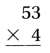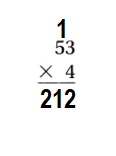Explanation:
Strategy used is multiplication and addition to find the product.
53 × 4 = (50 × 4) + (3 × 4)
= 200 + 12
= 212.

Question 2.Strategy used is multiplication and addition to find the product.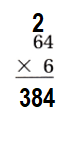Explanation:
64 × 6 = (60 × 6) + (4 × 6)
= 360 + 24
= 384.

Question 13.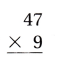Strategy used is multiplication and addition to find the product.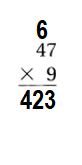Explanation:
47 × 9 = (40 × 9) + (7 × 9)
= 360 + 63
= 423.

Question 14.Strategy used is multiplication and addition to find the product.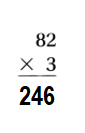Explanation:
82 × 3 = (80 × 3) + (2 × 3)
= 240 + 6
= 246.

Problem Solving
Use the table for 5-7.
Question 5.
The mayor of a town buys 4 packages of balloons for a street festival. How many balloons did the mayor buy?Total number of balloons the mayor buy = 232.

Explanation:
Number of packages of balloons for a street festival the mayor of a town buys = 4.
Number of balloons in each package = 58.
Total number of balloons the mayor buy = Number of packages of balloons for a street festival the mayor of a town buys × Number of balloons in each package
= 4 × 58
= (4 × 50) + ( 4 × 8)
= 200 + 32
= 232.

Question 6.
Roberto buys 4 packages of noisemakers and 3 packages of hats. Does he have more hats or more noisemakers? Explain.
He has 7 more hats than noisemakers.

Explanation:
Number of packages of noisemakers Roberto buys = 4.
Number of noisemakers  in each package = 17.
Total number of noisemakers Roberto buys = Number of packages of noisemakers Roberto buys  × Number of noisemakers  in each package
= 4 × 17
= (4 × 10) + (4 × 7)
= 40 + 28
= 68.
Number of packages of hats Roberto buys = 3.
Number of hats in each package =  25.
Total number of hats Roberto buys = Number of packages of hats Roberto buys × Number of hats in each package
= 3 × 25
= (3 × 20) + (3 × 5)
= 60 + 15
= 75.
Difference:
Total number of hats Roberto buys – Total number of noisemakers Roberto buys
= 75 – 68
= 7.

Question 7.
Nan buys 3 packages of party favors, If she has 100 people coming to a party, does she have enough favors? Explain.
No, she will not have enough favors to give to the people coming to a party.

Explanation:
Number of packages of party favors Nan buys = 3.
Number of favors in each package = 32.
Total number of favors Nan buys  = Number of packages of party favors Nan buys × Number of favors in each package
= 3 × 32
= (3 × 30) + (3 × 2)
= 90 + 6
= 96.
Number of people coming to a party = 100.
Difference:
Number of people coming to a party – Total number of favors Nan buys
= 100 – 96
= 4.

Question 8.
Erika has 6 boxes of buttons. Each box holds 28 buttons. Explain how Erika can use place value and regrouping to find how many buttons there are.
Total number of buttons Erika has = 168.

Explanation:
Number of boxes of buttons Erika has = 6.
Number of buttons each box holds = 28.
Total number of buttons Erika has =  Number of boxes of buttons Erika has × Number of buttons each box holds
= 6 × 28
Multiply 6 with the place value of 2 = 2 tens (2 ×10 = 20) and add to place value of 8 = ones (8 ×1 = 8)
= (6 × 20) + (6 × 8)
= 120 + 48
= 168.

Lesson Check
Texas Test Prep
Question 9.
It takes a train 42 minutes to ride ‘from Coltsville to Newburgh. If the train makes 8 trips in one day, how many minutes does it travel?
(A) 336 minutes
(B) 236 minutes
(C) 322 minutes
(D) 412 minutes
Number of minutes it travels = 336.
(A) 336 minutes.

Explanation:
Number of minutes a train takes to ride ‘from Coltsville to Newburgh = 42.
Number of trips a train takes in one day = 8.
Number of minutes it travels = Number of minutes a train takes to ride ‘from Coltsville to Newburgh  × Number of trips a train takes in one day
= 42 × 8
= (40 × 8) + (2 × 8)
= 320 + 16
= 336.

Question 10.
Charlie puts together boxes of model cars at a factory. If he packs 18 boxes in one hour, how many boxes does he pack in 7 hours?
(A) 187
(B) 156
(C) 76
(D) 126
Number of boxes he pack in 7 hours = 126.
(D) 126.

Explanation:
Number of boxes he packs in one hour = 18.
Number of boxes he pack in 7 hours = 7 × Number of boxes he packs in one hour
= 7 × 18
= (7 × 10) + (7 × 8)
= 70 + 56
= 126.

Question 11.
A bookstore has 6 shelves of art books. There are 27 books on each shelf. how many art hooks are in the bookstore?
(A) 33
(B) 44
(C) 112
(D) 162
Number of art books are in the bookstore = 162.
(D) 162.

Explanation:
Number of shelves of art books a bookstore has = 6.
Number of books on each shelf = 27.
Number of art books are in the bookstore = Number of shelves of art books a bookstore has × Number of books on each shelf
= 6 × 27
= (6 × 20) + (6 × 7)
= 120 + 42
= 162.

Question 12.
A deli has 6 boxes of soup cans. Each box holds 14 cans. How many soup cans does the deli have?
(A) 64
(B) 20
(C) 84
(D) 24
Number of soup cans the deli have = 84.
(C) 84.

Explanation:
Number of boxes of soup cans a deli has = 6.
Number of cans each box holds = 14.
Number of soup cans the deli have = Number of boxes of soup cans a deli has × Number of cans each box holds
= 6 × 14
= (6 × 10) + (6 × 4)
= 60 + 24
= 84.

Question 13.
Multi-Step Ashley has 4 bags each of red marbles and yellow marbles. Each bag holds 14 marbles. How many marbles does Ashley have?
(A) 56
(B) 112
(C) 39
(D) 92
Number of marbles Ashley have = 112.
(B) 112.

Explanation:
Number of bags of each red marbles and yellow marbles Ashley has = 4.
Number of marbles each bag holds = 14.
Number of marbles Ashley have = (2 × Number of bags of each red marbles and yellow marbles Ashley has ) × Number of marbles each bag holds
= (2 × 4) × 14
= 8 × 14
= (8 × 10) + (8 × 4)
= 80 + 32
= 112.

Question 14.
Multi-Step Devon collects 23 bottles and 4 boxes of 16 cans on recycling day. His goal is to collect 100 items. How many more items does Devon need to reach his goal?
(A) 43
(B) 39
(C) 87
(D) 13
Number of more items Devon needs to reach his goal = 13.
(D) 13.

Explanation:
Number of bottles Devon collects  = 23.
Number of boxes Devon collects  = 4.
Number of cans he collects = 16.
Number of items to collect his goal = 100.
Number of items he collected on recycling day = Number of bottles Devon collects + (Number of boxes Devon collects × Number of cans he collects)
= 23 + (4 × 16)
= 23 + 64
= 87.
Number of more items Devon needs to reach his goal = Number of items to collect his goal – Number of items he collected on recycling day
= 100 – 87
= 13.

Scroll to Top